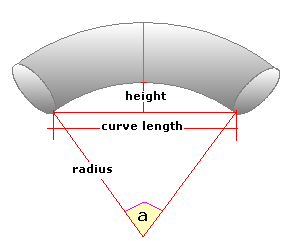Spike's CalculatorsCalculate the bend radius and the angle from the length of the curve and the height. This calculator uses inches for measurements. You do not always know the angle or the bend radius to calculate the volume of a curved section of pipe, but the two measurements needed for this calculation are easy to get.

Curve Length in
Curve Height in
Decimal Precision #

#### Calculator

1. enter the curve length in inches
2. the height of the curve in inches
3. decimal precision, the number of digits after the decimal point

#### Results

1. the bend radius in inches
2. the internal angle in radians
3. in decimal degrees
4. in degrees, minutes, and seconds

##### Formula
```r = (d²/h+h)/2
a = sin-1(d/r)
where r is the bend radius